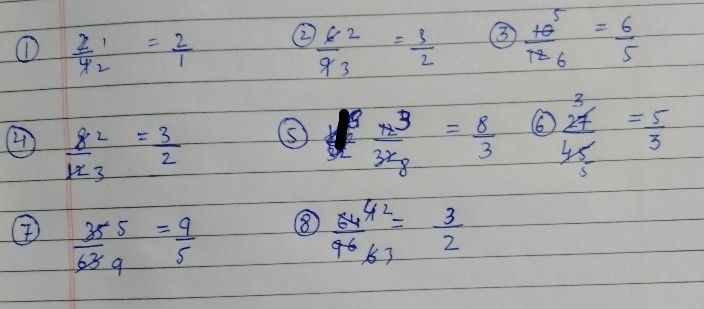Symbol
ProblemLearning Task $1$ Give the lowest term then write the reciprocal of the following fractions. Write your answer on your notebook. $1\right)$ $\dfrac {2} {4}$ $2\right)$ $\dfrac {6} {9}$ $3\right)$ $\dfrac {10} {12}$ $4\right)$ $\dfrac {8} {1}2$ $5\right)$ $\dfrac {12} {32}$ $6\right)$ $\dfrac {27} {45}$ $7\right)$ $\dfrac {35} {63}$ $8\right)$ $\dfrac {64} {96}$
1st-6th grade
Other
Search count: 115
Question content
please enter the correct answer
SolutionQanda teacher - Gulshan123Student
this is correct
I'm grade 5 student